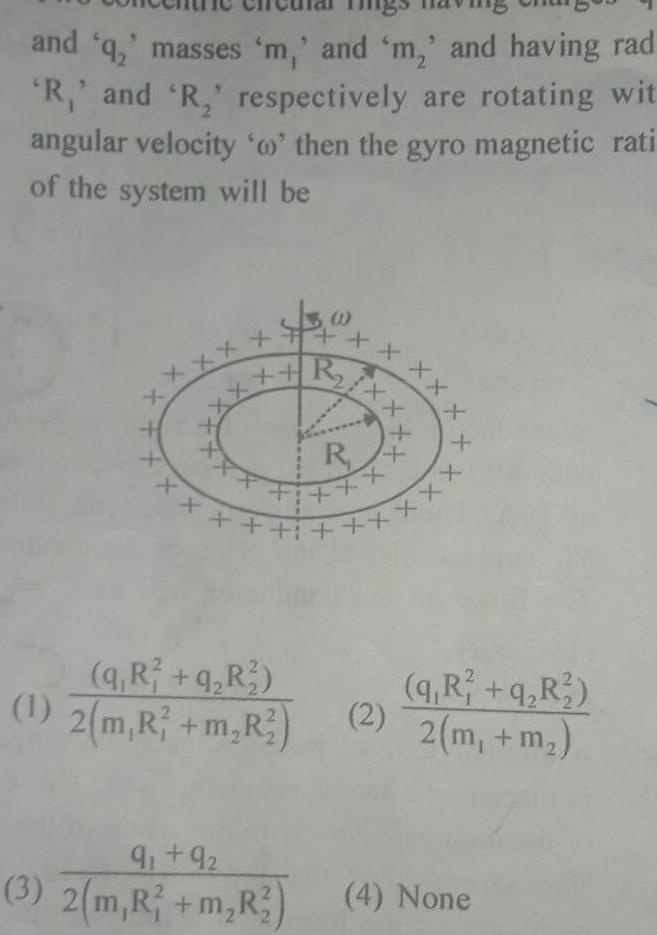Question:

# and q masses m and m and having rad R and R respectively are

Last updated: 6/2/2023and q masses m and m and having rad R and R respectively are rotating wit angular velocity o then the gyro magnetic rati of the system will be x 40 R q R q R 1 2 m R m R 9 92 3 2 m R m R2 x XX R XX i xxx q R q R 2 2 m m 4 None